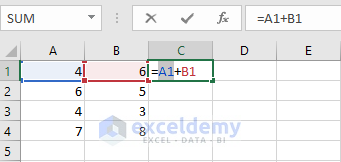Disclosure: This post may contain affiliate links, meaning when you click the links and make a purchase, we receive a commission.

# What is and How to Do Absolute Cell Reference in Excel?

In this article, I will discuss what is an absolute reference and how to do absolute reference in Excel? Then I will show how you can shift from one type of reference to another type.

Before understanding absolute cell references, you have to understand cell reference in a formula. Say I have a formula like this ‘=B3*C3+D3’. In this formula there are three cell references, they are B3, C3, and D3. Every reference in a formula links with a cell in excel worksheet. In another word, every cell has a unique reference. B3 can link to only one cell in the worksheet.

There are two types of references in excel-relative references and absolute references. Relative and absolute references behave differently when they are copied and filled with other cells. If you use relative references in a formula, the formula will change if you copy the formula to another cell. On the other hand, absolute references will remain constant, no matter in which they are copied.

## What is absolute cell references

Sometimes you may need that a cell reference will not change when you will fill cells. In relative references, when you copy your formula to another cell, the formula will be changed according to rows or columns. In contrast, in absolute references when you copy your formula to another cell, the whole formula will not change according to rows or columns.

Read More: Relative cell references in Excel

Say, for example, you have a formula like this ‘=D4*F2’ in cell E4. If E4 is active cell then click Fill Handle, hold it and drag over the cells you want to fill. See the following example. We have filled the cell ranges E5: E14 in our example. Check the formula in every cell. For example, we just double-click on cell E8 to check formula in it. You will find that the formula is ‘=D8*F6’. Row numbers have been increased by 4, D4 has been D8 and F2 has been F6. This is relative references.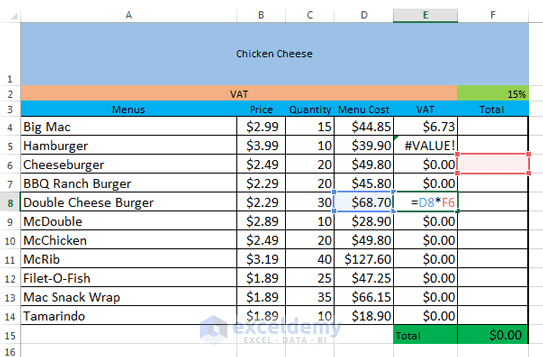A formula using relative cell references.

Read More: How to reference a cell from a different worksheet in Excel

## A formula using absolute references

But we want that F2 cell’s value will be unchanged, and its value will be as it is in the mother formula. For this, we have to use absolute references. We shall write newly our formula in cell E4 as ‘=D4*\$F\$2’. We have used a dollar sign(\$) before column letter and row number. This is the system how you can make a cell reference absolute.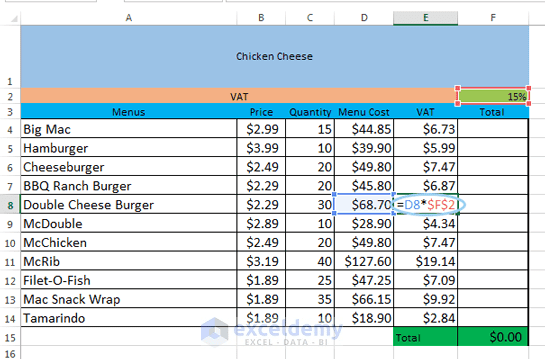Absolute cell references in Excel. Formula with absolute cell references.

## Three types of absolute references

There are three types of absolute references where you can make absolute the whole cell in a formula, only making the column absolute in a formula or only making the row absolute in a formula. The following table describes three types of absolute references.

### TABLE: Three types of absolute references

Absolute cell referencesDescription
\$F\$5Using these type of absolute references tell us that both column and row is fixed in the formula. It means that cell F5’s value is fixed in every formula in the filled cells.
F\$5Using this type of absolute references tell us that only row is fixed in the formula.
\$F5Using this type of absolute references tell us that only column is fixed in the formula.

Note: We shall mainly use \$F\$5 type of absolute reference in the formula. Last two types of absolute references are rarely used.

## Creating and Copying formula using absolute cell references in Excel

We shall create a formula in cell E4 to calculate the VAT for all items in column E. In our example the VAT rate is 15% and the value is set in cell F2. We shall use \$F\$2 absolute reference in our formula. As our every formula is using the same VAT rate, we want that reference will remain constant when the formula is copied and filled to other cells in column E.

Read More: Creating and Copying formula using relative references in Excel

### Step 1:

Select the cell that will contain your formula. In our example, the cell is E4 where we shall use the formula.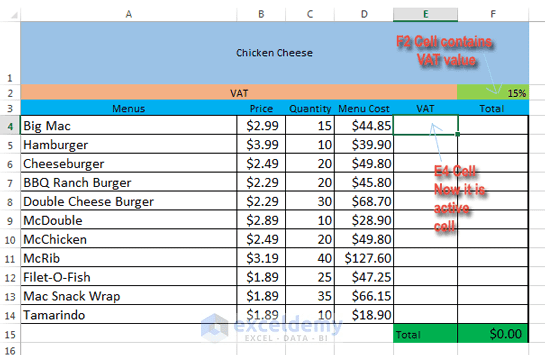Absolute cell references in Excel. E4 cell will contain the formula.

### Step 2:

Enter your formula to calculate the desired value in the selected cell. We shall type ‘=D4*\$F\$2’ in cell E4.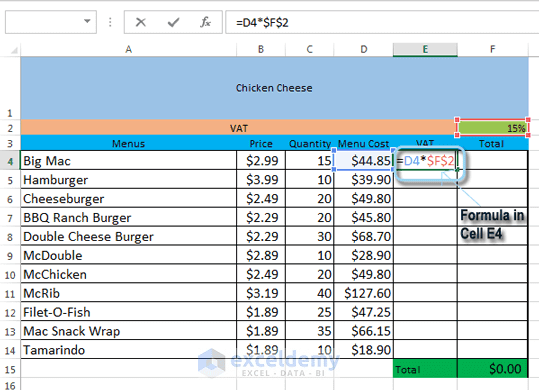Absolute cell references in Excel. E4 cell is containing ‘=D4*\$F\$2’ formula.

### Step 3:

Now press Enter on your keyboard. The formula will be calculated internally and you will see the calculated result in cell E4.

### Step 4:

By default when you pressed Enter, the active cell is now E5. Use up arrow(↑) key to make E4 as active cell again. Now locate the fill handle in the lower-right corner of the cell E4.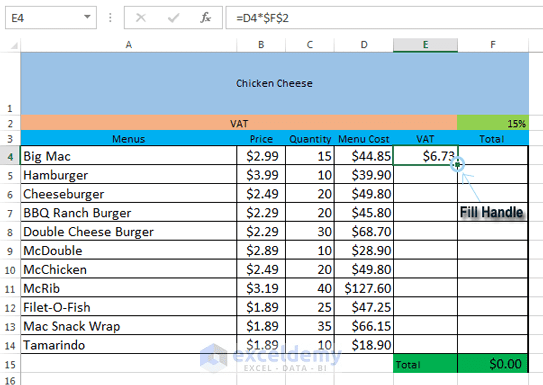Absolute cell references in Excel. Locating Fill Handle in cell E4.

### Step 5:

Now click the fill handle, hold it and drag over the cells you wish to fill. We are selecting cells E5: E14 in our example.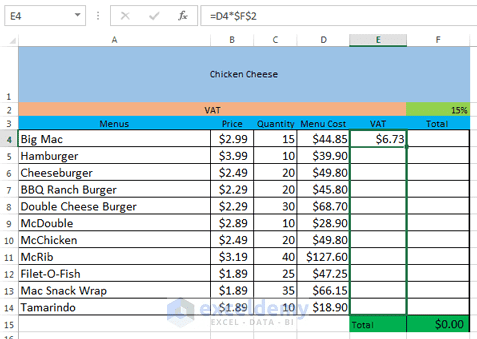Absolute cell references in Excel. Selecting cells dragging Fill Handle.

### Step 6:

Now release the mouse. The formula, we made in cell E4, will be copied to every cell we have selected with relative references. Calculated values will be visible in every cell.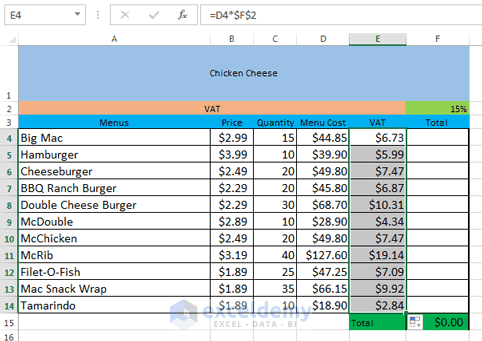Absolute cell references in Excel. Cells are filled with values.

Note: To check whether your formula is correct, just double-click on any cell from the filled cells. You will find that every formula has \$F\$2 absolute references, relative references have changed with the row numbers. For the row number 8, the formula is ‘=D8*\$F\$2’, just the row numbers have been changed.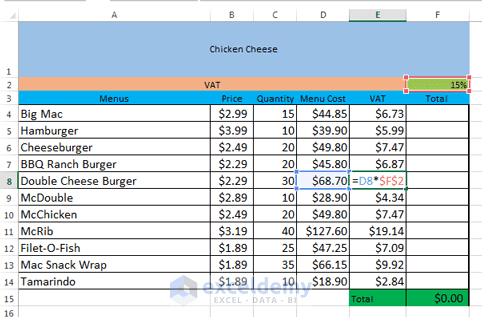Absolute cell references in Excel. The formula has both relative and absolute cell references. Relative references have changed with the row numbers but absolute cell references are same in every formula.

Read More: Excel Reference Cell in Another Sheet Dynamically

## Mixed Cell Reference in Excel

Mixed cell reference means either an absolute row and relative column or an absolute column and relative row. When we add the \$ sign before a row number, we create an absolute row or if we add the \$ sign before a column letter we create an absolute column.

In the following image, \$A1 is a mixed cell reference.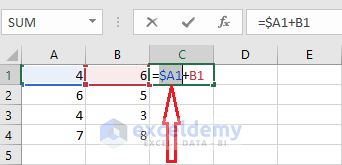## Switching between references

Typing \$ sign manually before the column letter and/or row number is very painful and boring work. I’m going to show here how you can shift a relative cell reference to absolute and then change absolute cell reference to mixed, and finally changing a mixed cell reference to a relative.

### 1) Making a cell reference absolute

Here I want to show you the shortcut way to make an absolute cell reference with an example.

I want to make A1 cell reference as an absolute cell reference in the cell C1.

At first, I take the C1 cell to edit mode. Now I keep the cursor on the A1 relative reference.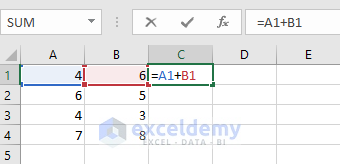When the cursor is on the A1 cell reference, I just press the F4 key on the keyboard. A1 cell reference will be converted into \$A\$1 absolute reference.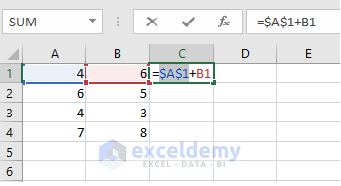### 2) Shifting an Absolute Cell Reference to Mixed Cell Reference

Continuing from above picture…

Now I press the F4 key again. \$A\$1 will be converted to a mixed cell reference: A\$1. The column will be relative but row number will be absolute.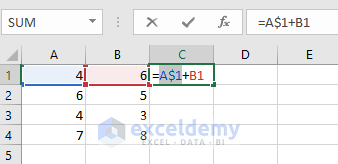Now if we again press the F4 function key again, then A\$1 will be converted into \$A1, that means Column letter will be absolute and row number will be relative.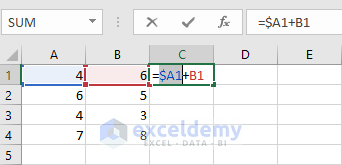### 3) Shifting a Mixed Cell Reference to Relative Cell Reference

Continuing from the above procedure……

Again if we press F4 function key again (Fourth times), then \$A1 will be converted into A1 relative cell reference.